# Catch up on the latest AI articles# Take Advantage Of Trained Models For Anomaly Detection Where Information Is Scarce.

3 main points
✔️ Achieve anomaly detection performance comparable to supervised learning as well as much better than SoTA
✔️ Achieve high accuracy with low computational cost by using features from existing learned models
✔️ Claimed that existing anomaly detection methods that try to learn features from normal data only have difficulty in acquiring features

The images used in this article are from the paper or created based on it.

## first of all

Among image recognition, abnormality detection, which determines whether an image is abnormal or not, is a task that has high applicability in the real world. For example, it can be used to select a small number of abnormal parts from a large number of normal parts produced in a factory.

However, due to the nature that what we want to detect is an abnormal image, there is almost always a data imbalance where there is little abnormal data compared to a large amount of normal data. Therefore, it is not possible to learn anomaly detection by the method in the normal image recognition task, and the task of anomaly detection has been studied independently. Such methods for anomaly detection have mostly proceeded with an approach to extract features of normal data from scratch, using only normal data. Simply put, if we are accustomed to seeing correct things in a state where we do not know anything about them, we may think something is suspicious when we see an anomaly.

However, the author of this paper disagreed with it. In this paper, we analyze the results of PCA-based based on the analysis of the results of principal component analysis. He argued that it is not possible to extract the features specific to normal data from scratch, which is the approach of existing methods for common anomaly detection.

Instead. We proposed that the features extracted by using the learned models of existing image classification methods should be used for anomaly detection. In other words, transfer learning in anomaly detection. In fact, the author achieves SOTA on the MVTec AD dataset by utilizing the learned models for anomaly detection. This approach may be a promising method that will allow us to effectively use the constantly evolving image recognition methods for anomaly detection.

## Outline of the proposed method

The flow of anomaly detection by the proposed method is as follows.

1. Normal data to be used for anomaly detection is input to the pre-trained model and inferred. EfficientNet (ImageNet's image recognition SOTA at the time of writing) trained on ImageNet is used as the pre-trained model.
2. The feature vectors extracted from the layers of the model after inference are approximated by a multivariate Gaussian distribution. We regard this as the data distribution of normal data.
3. The image to be inferred is input to the trained model, and the distance between the feature vector extracted from it and the distribution of normal data is calculated by Mahalanobis distance.
4. If the distance is more than a certain distance, it is identified as abnormal.

It can be said that the first two are the learning phase and the latter two are the inference phase, but since we are using a pre-trained model, the distribution of normal data is produced by approximating the inferred features to a distribution instead of learning. Extracting the features of normal data by diverting the existing learned model is the most important point of this paper, as mentioned above.

The approach of using learned models itself is not new, as it has been used frequently in other image recognition fields including transfer learning, but I personally think it is However, I personally think it is interesting from the viewpoint that it can be applied to other research. I think there are three points. I would like to share my personal opinion as well as the author's argument.

The first is that we do not perform any database-specific learning during anomaly detection.

As you can see from the flow of the proposed method, this anomaly detection is not trained with the dataset we want to detect anomalies. It only approximates the distribution of the results obtained by inputting the data to the trained model and inferring the results. Since it is a simple method without learning, it is quite attractive because of its low computational cost and fast computation time.

Secondly. the suggestion that the features used for discrimination in anomaly detection do not differ significantly within the normal class. The second suggestion is that the features used for identification in anomaly detection do not differ significantly within the normal class.

When I first read about it. I thought that the difference between the classes within normal data would exceed the difference between normal and abnormal data. This is because I thought there was a concern that the learned model would extract features to classify within normal data, even when used on normal data. After all, the learned model is trained to improve class classification performance using ImageNet and other methods. In particular, we thought that this could happen when there are multiple classes in normal data, even in the database for anomaly detection.

However, this seems to be an unfounded fear. The author examines why the features extracted by the pre-training model are valid.

The features used for identification in anomaly detection do not differ significantly within the normal class.

The authors use PCA to test this hypothesis. Specifically, we tested how the accuracy changes depending on the size of the variance of the components. As a result, we found that the principal component with the smallest variance is the one that is most used in anomaly detection among normal data.

The previous concern is a little bit in the wrong direction, but the variance may be due to differences between classes within normal data which is caused by the difference between classes in the normal data. It could be solved by saying that only features with small variance are effective for anomaly detection. If I'm missing the point of the question in the first place, please let me know.

The author also uses the results of this experiment to explain why it is not effective to learn normal data from scratch. It suggests that it is difficult to learn components with a small variance that are highly discriminative if we only learn from scratch without using prior learning.

## The mathematical background of model approximation

In this chapter, we describe the mathematical background at each step of the learning process. In addition to the methods for modeling the data during learning, we introduce the computational methods used for the metrics during inference and the proposed method for setting the threshold.

### Modeling and parameter estimation of normal data

In a nutshell, the way to model normal data is to approximate the probability density function of the normal class by the features obtained from the learned model, assuming that the features obtained from the learned model can be transferred to the task of anomaly detection. This can be regarded as a class-incremental learning method where new data classes are added gradually. The mathematical background used in the proposed method is as follows.

• In the proposed method, it is assumed that the feature vector x extracted by the trained model follows a multivariate Gaussian distribution (MVG). The multivariate Gaussian distribution is defined by the following equation, where D is the number of dimensions, µ is the mean vector and Σ is the positive definite covariance matrix.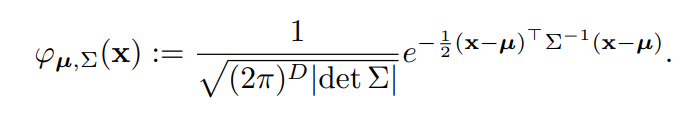• Since this is anomaly detection, the covariance matrix Σ of the actual normal data is unknown. Therefore, we perform parameter estimation, which is commonly done in statistics, and use the estimated value of Σ for common sense. In the observed data x1,. ,xn, the covariance matrix of the sample can be expressed as follows. However, the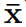is the sample mean vector, and n is the sample size.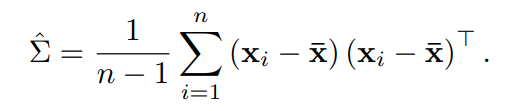• If the dimensionality D of the feature vector is sufficiently smaller than the sample size n, it is possible to substitute the above estimator into the multivariate Gaussian distribution formula. However, if D/n is not sufficiently small, the estimation result of the covariance matrix becomes unstable, and if D is sufficiently larger than n, the covariance matrix becomes singular and the inverse matrix cannot be calculated. The proposed method avoids this problem by using the Shrinkage method by Ledoit, Wolf et al. The Shrinkage method defines the new covariance matrix estimator is the empirical estimate of the covariance matrix plus the standardized unit matrix I. To what extent does the weighting parameter ρ define the amount of bias and variability trade-off? It is ρ that strikes a balance between using an estimator with high unbiased variability and high empirical value or using the value of the unit matrix with high bias and no variability. Ledoit and Wolf proposed that the optimal value of ρ can be calculated by minimizing the squared error between the post-shrunk estimator and the original estimator.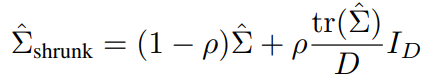### Method for determining abnormal values

In the proposed method, the anomaly is judged as an anomaly if the distance from the distribution is more than a certain value. The measure used in this method is Mahalanobis distance.

• The Mahalanobis distance represents the distance between a point (vector quantity) x and a Gaussian distribution with a mean of µ and covariance of Σ and is defined by the following equation The Mahalanobis distance is statistical in that it takes into account the correlation of the data and is independent of the scale level, and is easily used to calculate the uncertainty of the sample. It is also a type of distance that is easily used in the context of anomaly detection and for measuring outliers away from the distribution.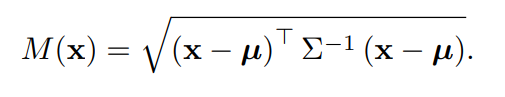We propose to derive the threshold value computationally, although the threshold value is generally determined empirically by human beings when we consider an outlier to be a certain distance from the distribution. First, the used We introduce the mathematical background.

• If x follows a multivariate Gaussian distribution, then M(x) 2 is known to follow a chi-square distribution with D degrees of freedom. Note that the chi-square distribution with k degrees of freedom is the sum of squares of k random variables, each of which follows an independent standard normal distribution. The probability density function of the chi-square distribution is shown in the equation below, where Γ(s) is called the gamma function and s>0.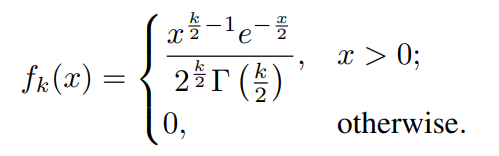• The cumulative distribution function of the chi-square distribution can be expressed as follows, where γ(s,x) is the incomplete gamma function.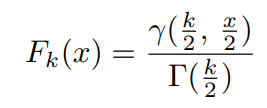Based on these properties, the author proposes a method to calculate the threshold value. Here, we use the fact that when a Mahalanobis distance coincides with the probability p of obtaining normal data in a chi-square distribution, this is what counts as the true negative rate (TNR ). Similarly, we believe that 1-p is consistent with the false positive rate (FPR). This idea is based on the fact that the Mahalanobis distance described above follows a chi-square distribution.

• According to this concept, the threshold value is set at point t, which is calculated using the value of the acceptable FPR and the cumulative distribution function of the chi-square distribution. This calculation of t is calculated from the properties of the chi-square distribution. t satisfies the following equation and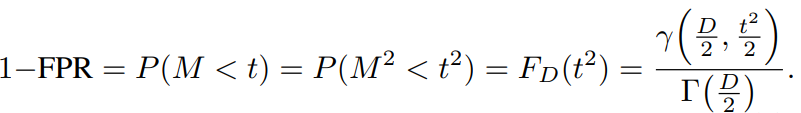• Based on this, t will be the following Here F D (x) is the cumulative distribution function described above.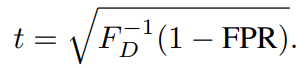By comparing the Mahalanobis distance between this threshold t and the feature vector of the image to be judged as abnormal, we can judge whether it is abnormal or not.

## Experimental results and discussion

In our experiments, we use EfficientNet, which was the SOTA for image identification in ImageNet at the time of writing, and ResNet, which is easily used for comparison, as the pre-training model.

The following experiments have been conducted to check the validity of each parameter and method

1. Evaluation of the selection of feature extraction layers
2. Evaluating methods for calculating the distribution and anomaly distances to be assumed
3. Comparison of pre-training models
4. Evaluating the different scales of EfficientNet.
5. Evaluation of the validity of the threshold

In addition to the validity of these methods, we also evaluate their performance in comparison with existing anomaly detection methods and discuss why they work. The latter is discussed in the Interest section of this paper. In this chapter, we review the performance experiments and their evaluations in comparison with other anomaly detection methods.

### Performance evaluation based on comparison with existing anomaly detection methods

In this paper, we compare our method with several anomaly detection algorithms, including SOTA's method on the MVTec AD dataset. Specifically, we compare the results with a semi-supervised reconstruction method using convolutional AE, a supervised AD classifier, oc-SVM using pre-trained features in EfficientNet, and the currently proposed SOTA method in the MVTec paper. Although the supervised classifier cannot be practically used for unlabeled anomaly detection, we benchmark it as an upper bound of what anomaly detection can produce.

The results of the comparison with each of these methods are shown below. As you can see, the proposed method, which uses multivariate Gaussian distribution for pre-trained features, achieves 10% higher average AUROC than SPADE, which is the next best method. It should be noted that even SPADE uses pre-trained features for k-NN and L2 Loss, which shows the usefulness of pre-trained features. Furthermore, the Fine-tuned pre-trained supervised classifier is comparable to the benchmark as an upper limit of performance. Incidentally, the supervised classifier is outperformed especially in the texture (medicine, screw) category.

In addition, the NPCA used here is the result of the PCA analysis that was used to verify why the method works. Specifically, negated PCA (NPCA) is the use of the principal components of the features that have the least variance left. By slightly increasing the robustness of the result method using this, the AUROC value is increased and the SEM value is decreased.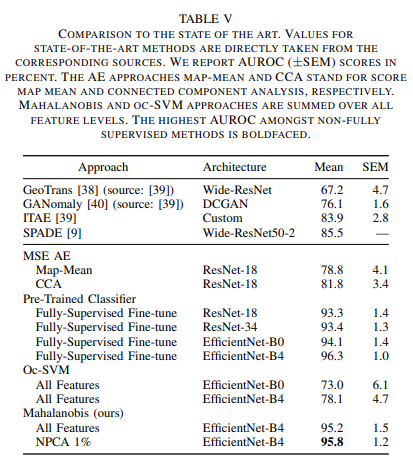## summary

By using the learned model for anomaly detection, the author achieves a level of performance comparable to that of normal supervised learning. The author also argues against the usual method of anomaly detection, which is to learn only from the normal, and argues that we should rather consider how to transfer the normal supervised learning. To do so, we would like to deepen our research on how to increase the generality of features, how to fine-tune the transferred features with a small amount of data, and how to utilize them in a multimodal data distribution.

As this approach is further researched, it may be a highly promising method to make effective use of constantly developing image recognition methods for anomaly detection.

If you have any suggestions for improvement of the content of the article,